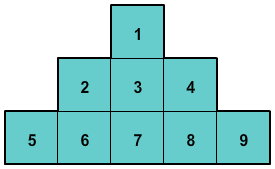# Area of Figures Formed with Complete Unit Squares

Rate 0 stars Common Core
Quiz size:
Message preview:
Someone you know has shared quiz with you:

To play this quiz, click on the link below:

https://www.turtlediary.com/quiz/area-of-figures-formed-with-complete-unit-squares.html

Hope you have a good experience with this site and recommend to your friends too.

Login to rate activities and track progress.
Login to rate activities and track progress.

A unit square is a square having each side of length 1 unit. The area of a unit square is 1 square unit.

For example, consider the following figure made up of unit squares.Let's find the area of this figure.

To find out the area of the given figure, we need to count the total number of unit squares.There are 9 unit squares inside the given figure.

So, the area of the given figure is 9 square units.

ds

A B C D E F G H I J K L M N O P Q R S T U V W X Y Z